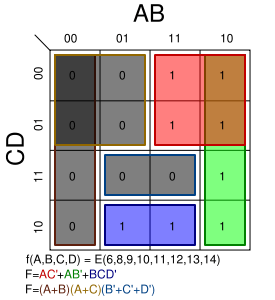# Karnaugh map – 카노 맵The Karnaugh map, also known as a Veitch diagram (K-map or KV-map for short), is a tool to facilitate management of Boolean algebraic expressions. A Karnaugh map is unique in that only one variable changes value between squares, in other words, the rows and columns are ordered according to the principles of Gray code.

Usage in boolean logic
Normally, extensive calculations are required to obtain the minimal expression of a Boolean function, but one can use a Karnaugh map instead.

* Problem solving uses
– Karnaugh maps make use of the human brain’s excellent pattern-matching capability to decide which terms should be combined to get the simplest expression.
– K-maps permit the rapid identification and elimination of potential race hazards, something that boolean equations alone cannot do.
– A Karnaugh map is an excellent aid for simplification of up to six variables, but with more variables it becomes hard even for our brain to discern optimal patterns.
– For problems involving more than six variables, solving the boolean expressions is more preferred than the Karnaugh map.
Karnaugh maps also help teach about Boolean functions and minimization.

Properties

A mapping of minterms on a Karnaugh mapA Karnaugh map may have any number of variables, but usually works best when there are only a few – between 2 and 6 for example. Each variable contributes two possibilities to each possibility of every other variable in the system. Karnaugh maps are organized so that all the possibilities of the system are arranged in a grid form and between two adjacent boxes only one variable can change value. This is what allows it to reduce hazards.

When using a Karnaugh map to derive a minimized function, one “covers” the ones on the map by rectangular “coverings” that contain a number of boxes equal to a power of 2 (for example, 4 boxes in a line, 4 boxes in a square, 8 boxes in a rectangle, etc). Once a person has covered the ones, a term of a sum of products is produced by finding the variables that do not change throughout the entire covering, and taking a 1 to mean that variable and a 0 as the complement of that variable. Doing this for every covering gives you a matching function.

One can also use zeros to derive a minimized function. The procedure is identical to the procedure for ones except that each term is a term in a product of sums – and a 1 means the complement of the variable while 0 means the variable non-complemented.

Each square in a Karnaugh map corresponds to a minterm (and maxterm). The picture to the right shows the location of each minterm on the map.

Map
The input variables can be combined in 16 different ways, so our Karnaugh map has to have 16 positions. The most convenient way to arrange this is in a 4×4 grid.K-map showing minterms and boxes covering the desired mintermsThe binary digits in the map represent the function’s output for any given combination of inputs. We write 0 in the upper leftmost corner of the map because f = 0 when A = 0, B = 0, C = 0, D = 0. Similarly we mark the bottom right corner as 1 because A = 1, B = 0, C = 1, D = 0 gives f = 1. Note that the values are ordered in a Gray code, so that precisely one variable flips between any pair of adjacent cells.

After the Karnaugh map has been constructed our next task is to find the minimal terms to use in the final expression. These terms are found by encircling groups of 1’s in the map. The encirclings must be rectangular and must have an area that is a power of two (i.e. 1, 2, 4, 8, …). The rectangles should be as large as possible without containing any 0’s. The optimal encirclings in this map are marked by the green, red and blue lines.

For each of these encirclings we find those variables that have the same state in each of the fields in the encircling. For the first encircling (the red one) we find that:

– The variable A maintains the same state (1) in the whole encircling, therefore it should be included in the term for the red encircling.
– Variable B does not maintain the same state (it shifts from 1 to 0), and should therefore be excluded.
– C does not change: it is always 0.
– D changes.
Thus the first term in the Boolean expression is AC’.

For the green encircling we see that A and B maintain the same state, but C and D change. B is 0 and has to be negated before it can be included. Thus the second term is AB’.

In the same way, the blue rectangle gives the term  BCD’ and so the whole expression is: AC’ + AB’ + BCD’.

출처: 위키사전